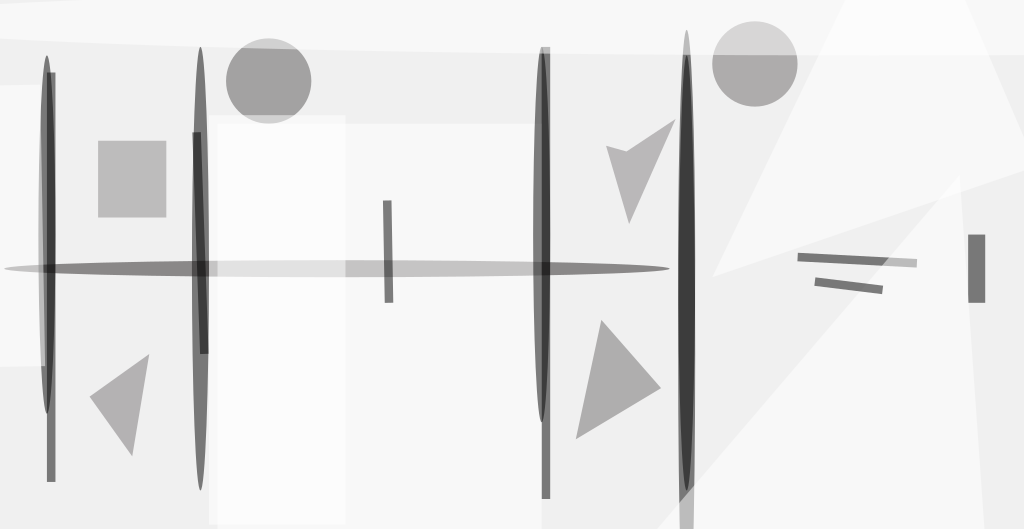A proposed design for a phased array fed cylindrical reflector microwave antenna would enable enhancement of the radiation pattern through partially adaptive amplitude and phase control of its edge radiating feed elements. Antennas based on this design concept would be attractive for use in radar (especially synthetic aperture radar) and other systems that could exploit electronic directional scanning and in which there are requirements for specially shaped radiation patterns, including ones with low side lobes. One notable advantage of this design concept is that the transmitter/receiver modules feeding all the elements except the edge ones could be identical and, as a result, the antenna would cost less than in the cases of prior design concepts in which these elements may not be identical.

The basic antenna geometry (see figure) is that of a parabolic cylindrical reflector. The cylindrical axis is the y axis, and the geometric boresight axis is the z axis. The phased array of feed elements lies on the focal line, which is parallel to the y axis, at a distance F from the apex of the parabola. The boundary of the reflector and thus of the aperture, as projected onto the x,y plane, is specified by the superquadric curvewhere a and b are the lengths of the x and y semiaxes, respectively, of the aperture; and m is a parameter that can be chosen to control the rounded shape of the corners. These basic geometric characteristics are the same as those of the antenna described in "Low-Sidelobe Phased-Array-Fed Cylindrical-Reflector Antenna (NPO-20494), NASA Tech Briefs, Vol. 24, No. 2 (February 2000), page 5a.

For the non-edge radiating elements, the amplitudes may be chosen to obtain suitably shaped main and side lobes, and the phase difference between adjacent elements would be set at the value needed to point the main lobe in the desired direction. For the nth non-edge element, the excitation coefficient would be of the form

ane jnα,

where an is the magnitude of the excitation, and a is the progressive phase (that is, the phase difference between adjacent elements) needed to point the main lobe of the radiation beam in a given direction in the (y,z) plane. For an edge radiating element, the excitation coefficient would be of the form

we = a±N/2e±jNα/2(1+ce±jδ),

where w and a±N/2 are magnitudes;Φ is the phase of the excitation; c and δ are a magnitude scale factor and a phase shift, respectively, necessary for the desired degree of cancellation of a specified side lobe, and N is one less than the total number of radiating elements.

Computational simulations have been performed, following diffraction-analysis procedures based on a physical-optics formulation. Some of the parameters used in the simulations were a = 5.4 m, b = 3 m, F = 4.32 m, and N + 1 (the number of elements) = 41. The phased array had a length equal to that of the cylindrical axis of the antenna (2b = 6 m) and comprised 41 y-polarized elements spaced at intervals of 0.63 wavelength. The results of the computational simulations showed that the radiation pattern could be controlled with high versatility through control of the edge-element excitation amplitudes and phases. In particular, it was demonstrated that the side-lobe levels could be reduced (see Figure 2) and even effectively canceled and that side-lobe envelopes could be made steeper (side-lobe levels made lower) by choosing, for cancellation, a specific side lobe near the peak of the main lobe. In addition, one could choose the edge-element excitations to obtain different side-lobe envelopes simultaneously on the opposite sides of the main lobe.

This work was done by Ziad Hussein and Jeff Hilland of Caltech for NASA's Jet Propulsion Laboratory. For further information, access the Technical Support Package (TSP) free on-line at www.techbriefs.com/tsp under the Computers/Electronics category.

NPO-30251

This Brief includes a Technical Support Package (TSP).